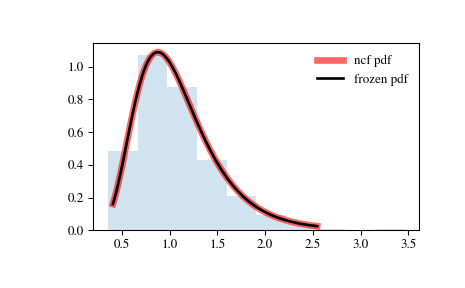# scipy.stats.ncf¶

scipy.stats.ncf = <scipy.stats._continuous_distns.ncf_gen object>[source]

A non-central F distribution continuous random variable.

As an instance of the rv_continuous class, ncf object inherits from it a collection of generic methods (see below for the full list), and completes them with details specific for this particular distribution.

Notes

The probability density function for ncf is:

$\begin{split}f(x, n_1, n_2, \lambda) = \exp(\frac{\lambda}{2} + \lambda n_1 \frac{x}{2(n_1 x+n_2)}) n_1^{n_1/2} n_2^{n_2/2} x^{n_1/2 - 1} \\ (n_2+n_1 x)^{-(n_1+n_2)/2} \gamma(n_1/2) \gamma(1+n_2/2) \\ \frac{L^{\frac{v_1}{2}-1}_{v_2/2} (-\lambda v_1 \frac{x}{2(v_1 x+v_2)})} {(B(v_1/2, v_2/2) \gamma(\frac{v_1+v_2}{2})}\end{split}$

for $$n_1 > 1$$, $$n_2, \lambda > 0$$. Here $$n_1$$ is the degrees of freedom in the numerator, $$n_2$$ the degrees of freedom in the denominator, $$\lambda$$ the non-centrality parameter, $$\gamma$$ is the logarithm of the Gamma function, $$L_n^k$$ is a generalized Laguerre polynomial and $$B$$ is the beta function.

ncf takes df1, df2 and nc as shape parameters.

The probability density above is defined in the “standardized” form. To shift and/or scale the distribution use the loc and scale parameters. Specifically, ncf.pdf(x, dfn, dfd, nc, loc, scale) is identically equivalent to ncf.pdf(y, dfn, dfd, nc) / scale with y = (x - loc) / scale.

Examples

>>> from scipy.stats import ncf
>>> import matplotlib.pyplot as plt
>>> fig, ax = plt.subplots(1, 1)


Calculate a few first moments:

>>> dfn, dfd, nc = 27, 27, 0.416
>>> mean, var, skew, kurt = ncf.stats(dfn, dfd, nc, moments='mvsk')


Display the probability density function (pdf):

>>> x = np.linspace(ncf.ppf(0.01, dfn, dfd, nc),
...                 ncf.ppf(0.99, dfn, dfd, nc), 100)
>>> ax.plot(x, ncf.pdf(x, dfn, dfd, nc),
...        'r-', lw=5, alpha=0.6, label='ncf pdf')


Alternatively, the distribution object can be called (as a function) to fix the shape, location and scale parameters. This returns a “frozen” RV object holding the given parameters fixed.

Freeze the distribution and display the frozen pdf:

>>> rv = ncf(dfn, dfd, nc)
>>> ax.plot(x, rv.pdf(x), 'k-', lw=2, label='frozen pdf')


Check accuracy of cdf and ppf:

>>> vals = ncf.ppf([0.001, 0.5, 0.999], dfn, dfd, nc)
>>> np.allclose([0.001, 0.5, 0.999], ncf.cdf(vals, dfn, dfd, nc))
True


Generate random numbers:

>>> r = ncf.rvs(dfn, dfd, nc, size=1000)


And compare the histogram:

>>> ax.hist(r, density=True, histtype='stepfilled', alpha=0.2)
>>> ax.legend(loc='best', frameon=False)
>>> plt.show()Methods

 rvs(dfn, dfd, nc, loc=0, scale=1, size=1, random_state=None) Random variates. pdf(x, dfn, dfd, nc, loc=0, scale=1) Probability density function. logpdf(x, dfn, dfd, nc, loc=0, scale=1) Log of the probability density function. cdf(x, dfn, dfd, nc, loc=0, scale=1) Cumulative distribution function. logcdf(x, dfn, dfd, nc, loc=0, scale=1) Log of the cumulative distribution function. sf(x, dfn, dfd, nc, loc=0, scale=1) Survival function (also defined as 1 - cdf, but sf is sometimes more accurate). logsf(x, dfn, dfd, nc, loc=0, scale=1) Log of the survival function. ppf(q, dfn, dfd, nc, loc=0, scale=1) Percent point function (inverse of cdf — percentiles). isf(q, dfn, dfd, nc, loc=0, scale=1) Inverse survival function (inverse of sf). moment(n, dfn, dfd, nc, loc=0, scale=1) Non-central moment of order n stats(dfn, dfd, nc, loc=0, scale=1, moments=’mv’) Mean(‘m’), variance(‘v’), skew(‘s’), and/or kurtosis(‘k’). entropy(dfn, dfd, nc, loc=0, scale=1) (Differential) entropy of the RV. fit(data, dfn, dfd, nc, loc=0, scale=1) Parameter estimates for generic data. expect(func, args=(dfn, dfd, nc), loc=0, scale=1, lb=None, ub=None, conditional=False, **kwds) Expected value of a function (of one argument) with respect to the distribution. median(dfn, dfd, nc, loc=0, scale=1) Median of the distribution. mean(dfn, dfd, nc, loc=0, scale=1) Mean of the distribution. var(dfn, dfd, nc, loc=0, scale=1) Variance of the distribution. std(dfn, dfd, nc, loc=0, scale=1) Standard deviation of the distribution. interval(alpha, dfn, dfd, nc, loc=0, scale=1) Endpoints of the range that contains alpha percent of the distribution

scipy.stats.ncx2

scipy.stats.nct